### Home > CALC > Chapter 4 > Lesson 4.4.3 > Problem4-151

4-151.
1. Define possible functions f(x) and g(x) so that h(x) = f(g(x)). Remember f(x) ≠ x, and g(x) ≠ x. Homework Help ✎

1.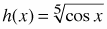2. h(x) = (3x cos(x2))3

3. h(x) = 1

4. h(x) = x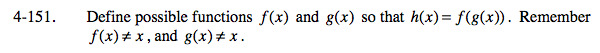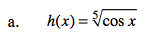f(x) =_____________
g(x) = cosx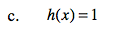One possible solution:
f(x) = x0
g(x) = x2
Find another.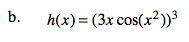One possible solution:
f(x) = x³
g(x) = 3xcos(x²)
Find another.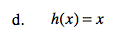Recall the definition of inverse functions:
f(x) and g(x) are inverse functions if f(g(x)) = x